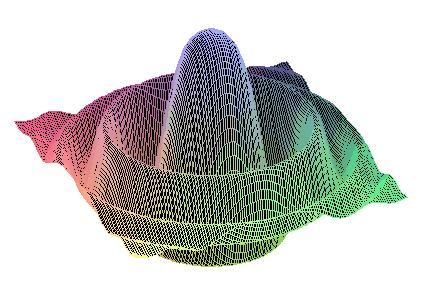DIANA Research Group
DIfferential Algebras and Nonlinear Analysis

Algebras of Generalized Functions

 GF2009    Home    People    Research      Research Topics      Research Projects      START PROJECT      Related Research    Teaching      Current Teaching      Diploma Theses
 The theory of algebras of generalized functions has been initiated by J.F.Colombeau and others about 20 years ago. Since then it has taken up rapid development and has found numerous applications in different fields of mathematics and mathematical physics. These include nonlinear PDE, distribution theory, nonsmooth differential geometry, nonlinear theory of stochastic processes, symmetries of differential equations, nonstandard analysis, quantum field theory, theory of relativity,... One of the starting points for the development of algebras of generalized functions has been the problem of multiplication of distributions. By a famous result of L.Schwartz, it is impossible to define an intrinsic multiplication on the space of distributions compatible with pointwise multiplication of continuous functions. However, one can construct differential algebras containing spaces of distributions as linear subspaces and the space of smooth functions as a faithful subalgebra while at the same time possessing optimal permanence properties concerning differentiation. Multiplication of distributions can be handled in this framework. Today, however, the theory of algebras of generalized functions reaches far beyond this original task. One reason for it's wide applicability lies in the direct and natural approach it takes towards introducing nonlinear concepts into distribution theory: Key notions of this approach are regularization processes for gaining smooth representatives of singular objects and factorization of differential algebras in order to obtain satisfying structures. Both concepts are basic constructions of many branches of modern mathematics. Monographs on the nonlinear theory of generalized functions.
Last changed January 3, 2007 by M.K. and R.S.